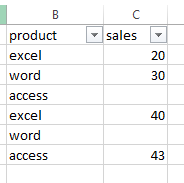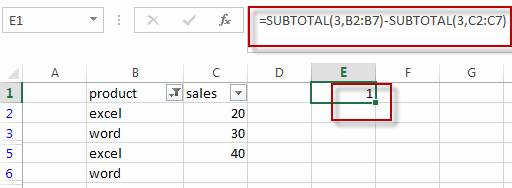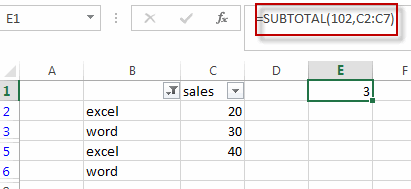# Count Blank or Non-blank Cells in Filtered Range

This post will guide you how to count blank or empty cells in a filtered range of cells in excel. How do I count non-blank cells in a filtered range of cells in excel. How to count blank or non-blank cells in a filtered list with SUBTOTAL function in a worksheet.

## Count Blank Cells

If you want to count only the visible blank cells in a filtered list in your worksheet, you can use a formula based on the SUBTOTAL function. For example, you have a filtered range of cells B1:C7, and those two columns have been filtered.And you want to get the number of all visible blank cells in column C. you just need to use the following formula:

`=SUBTOTAL(3,B2:B7)-SUBTOTAL(3,C2:C7)`Type this formula into a blank cell and then press Enter key in your keyboard. You will see the number of all visible blank cells in your current filtered cells.

## Count non-blank Cells

If you want to count the number of all visible non-blank cells in a filtered range, you can use the following formula based on the SUBTOTAL function.

`=SUBTOTAL(102,C2:C5)`### Related Functions

• Excel SUBTOTAL function
he Excel SUBTOTAL function returns the subtotal of the numbers in a list or database. The syntax of the SUBTOTAL function is as below:= SUBTOTAL (function_num, ref1, [ref2])….
Related Posts

Free Family Budget Template (Step-by-step Guide)

This article will show you some free printable family budget templates for google sheet and Microsoft Excel spreadsheet applications and will also explain some of the features or important functions of these templates. This will make it easy for you ...

Sort/Rank Numeric Values with Duplicate Values Exist

Excel built-in RANK function can sort a set of values. If there are duplicate numbers, then the rank number is also duplicated. See the following example: There are two numbers “100” in range A2:A9, they are both the third largest ...

Calculate Days Open in Excel

If you want to know how to Calculate days in Excel, there are some formulas that you can use to do so. For example, you can use the DAYS function in Excel to find the number of days between two ...

Calculate Grades With VLookup in Excel

Why Should You Calculate Grades With VLookup Excel? If you're looking for a simple way to find out a student's grades, VLOOKUP Excel can do the trick. This function uses a lookup table to find the values and sort them ...

Repeating Character n Times in Excel

In daily life, we can use repeated characters to indicate the magnitude or priority of something. For example, a single “!” indicates a minor case, double “!!” indicates a medium case, and triple “!!!” indicates a high case, and so ...

Sidebar